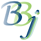# NUM() Function - BBj

For this topic's original documentation, see NUM() Function - Convert String to Numeric.

## Syntax

NUM(objexpr{,ERR=lineref})

## Description

The BBj NUM() function converts an object expression to a numeric equivalent.

 Parameter Description objexpr Value to be converted to a number. The value must be a number or a string. ERR=lineref Branch to be taken if an error occurs during execution.

## Remarks

When processing numeric input with INPUT X, and when converting a string to a number with NUM("123.45"), BBj only recognizes "." as the decimal separator. Many countries use "," as the decimal separator, with keyboards configured for that preference. In BBj 19 and higher, the ALLOW_DECIMAL_COMMA !COMPAT setting can be set to TRUE to have the INPUT verb and NUM()function accept both "." and "," as valid decimal separators.

## Example 1

NUM() is legal if the object expression evaluates to a number:

 >num!=123 >?num(num!) 123

## Example 2

NUM() is legal if the object expression evaluates to a string.

 >num!="123" >?num(num!) 123

## Example 3

NUM() throws an error if the object function does not evaluate to a number or string.

 >num!=new java.lang.Integer("123") >?num(num!)!ERROR=26 (Invalid number)